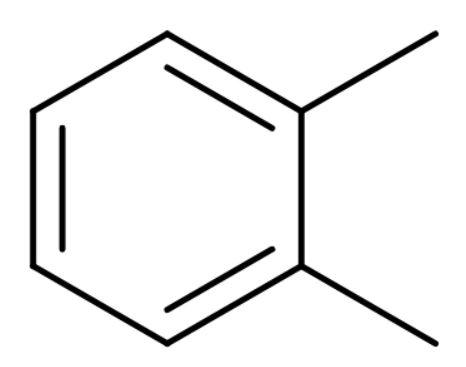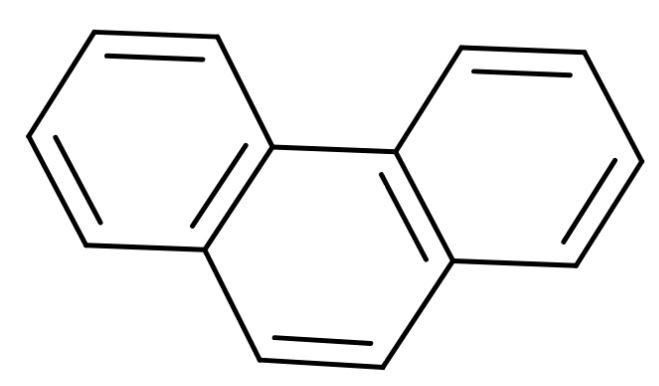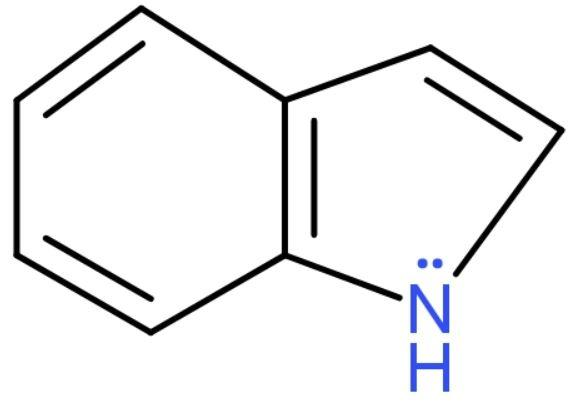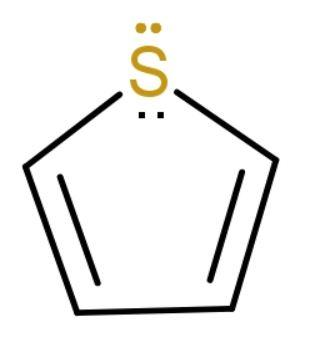Which among the following is a non-benzenoid aromatic compound?A. o-xyleneB. PhenanthreneC. IndoleD. ThiopheneVerified
146.4k+ views
Hint:Aromaticity means a conjugated system made of alternating single and double bonds in a ring. This conjugation allows the electrons in the molecule to be delocalized around the ring thus, increasing the molecule's stability.

To find the answer to this question, we should know what non-benzenoid compounds are, these are aromatic compounds which have highly unsaturated rings other than benzene rings. Aromatic compounds are cyclic and planar structures with a ring of resonance bonds. Aromatic molecules are very stable. Let us discuss the options one by one to find non-benzenoid aromatic compound:
A. o-xylene: It is also known as ortho-xylene is an aromatic compound with the formula ${{\text{C}}_{6}}{{\text{H}}_{4}}{{\left( \text{C}{{\text{H}}_{3}} \right)}_{2}}$. The two methyl groups bonded to adjacent carbon atoms of a benzene ring. It is an isomer of m-xylene and p-xylene and their mixture is called xylene or xylenes. Its structure isBy structure it is clear that it is a benzenoid compound.
B. Phenanthrene: A polycyclic aromatic compound composed of three fused benzene rings. It is made up of phenyl and anthracene. Its structure isAs it is a fusion of benzene rings then, it is a benzenoid compound.
C. Indole: Its molecular formula is ${{\text{C}}_{8}}{{\text{H}}_{7}}\text{N}$ and it is an aromatic heterocyclic organic compound. Its structure is bicyclic consisting of a six-membered benzene ring attached to a five-membered pyrrole ring. Its structure isThe lone pair on nitrogen is in resonance with the rings and satisfies Huckel’s Rule (the compound should have $\left( 4\text{n}+\text{2} \right)\pi \text{ }{{\text{e}}^{-}}$ in it). This compound has 10 $\pi {{\text{e}}^{-}}$in it which satisfies the rule. It has benzene attached to it. So, it is a benzenoid aromatic compound.
D. Thiophene: Thiophene is aromatic, heterocyclic compound with molecular formula ${{\text{C}}_{4}}{{\text{H}}_{4}}\text{S}$. Consisting of a planar five-membered ring. It resembles benzene. The lone pair on sulphur is delocalized in the pi electron system. Its structure isIt has 6 $\pi {{\text{e}}^{-}}$ inside the ring. It is aromatic but it is not attached to any benzene ring. So, it is a non-benzenoid compound.

The correct answer to this question is option ‘d’ (Thiophene).

Note:
By using Huckel’s Rule, we can check whether a compound is aromatic or not. The conditions of aromaticity are (i) should have $\left( 4\text{n}+\text{2} \right)\pi \text{ }{{\text{e}}^{-}}$in it. (ii) should have cyclic and planar structure and (iii) the electrons present should be inside the ring only.